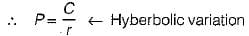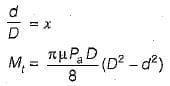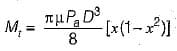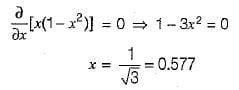# Test: Friction Clutches - 1

## 10 Questions MCQ Test Topicwise Question Bank for Mechanical Engineering | Test: Friction Clutches - 1

Description
Attempt Test: Friction Clutches - 1 | 10 questions in 30 minutes | Mock test for Mechanical Engineering preparation | Free important questions MCQ to study Topicwise Question Bank for Mechanical Engineering for Mechanical Engineering Exam | Download free PDF with solutions
QUESTION: 1

Solution:
QUESTION: 2

Solution:
QUESTION: 3

### Which of the followings is not a friction clutch?

Solution:
QUESTION: 4

Which of the following is not a characteristic of a positive contact clutch?

Solution:

A positive contact clutch does not develop heat appreciably during use.

QUESTION: 5

The clutch used in farm tractors and machine tools

Solution:
QUESTION: 6

The frictional torque transmitted by a disc clutch, considering uniform pressure, is the same as that for a

Solution:
QUESTION: 7

The pressure distribution over the entire surface area of the friction disk according to uniform wear theory is illustrated in

Solution:

As per uniform wear theory,
Pr - constantQUESTION: 8

The torque transmitting capacity of a friction clutch can be increased by following methods except

Solution:

Concept: The torque transmitting capacity of friction clutches is given by

T = μ W Rm

Now the torque transmitting capacity can be increased by

• Increasing μ (coefficient of friction)
• Increasing W (pressure on plate)
QUESTION: 9

A single pole clutch consists of only one pair of contacting surfaces. Assuming uniform wear theory, the torque transmitting capacity of the clutch is maximum when (d/D) is equal to

Solution:(Assuming uniform wear theory)for maximum Mt,QUESTION: 10

Select the wrong statement:

Solution:

A major portion of the life of friction lining comes under uniform wear criterion.Use Code STAYHOME200 and get INR 200 additional OFF Use Coupon Code